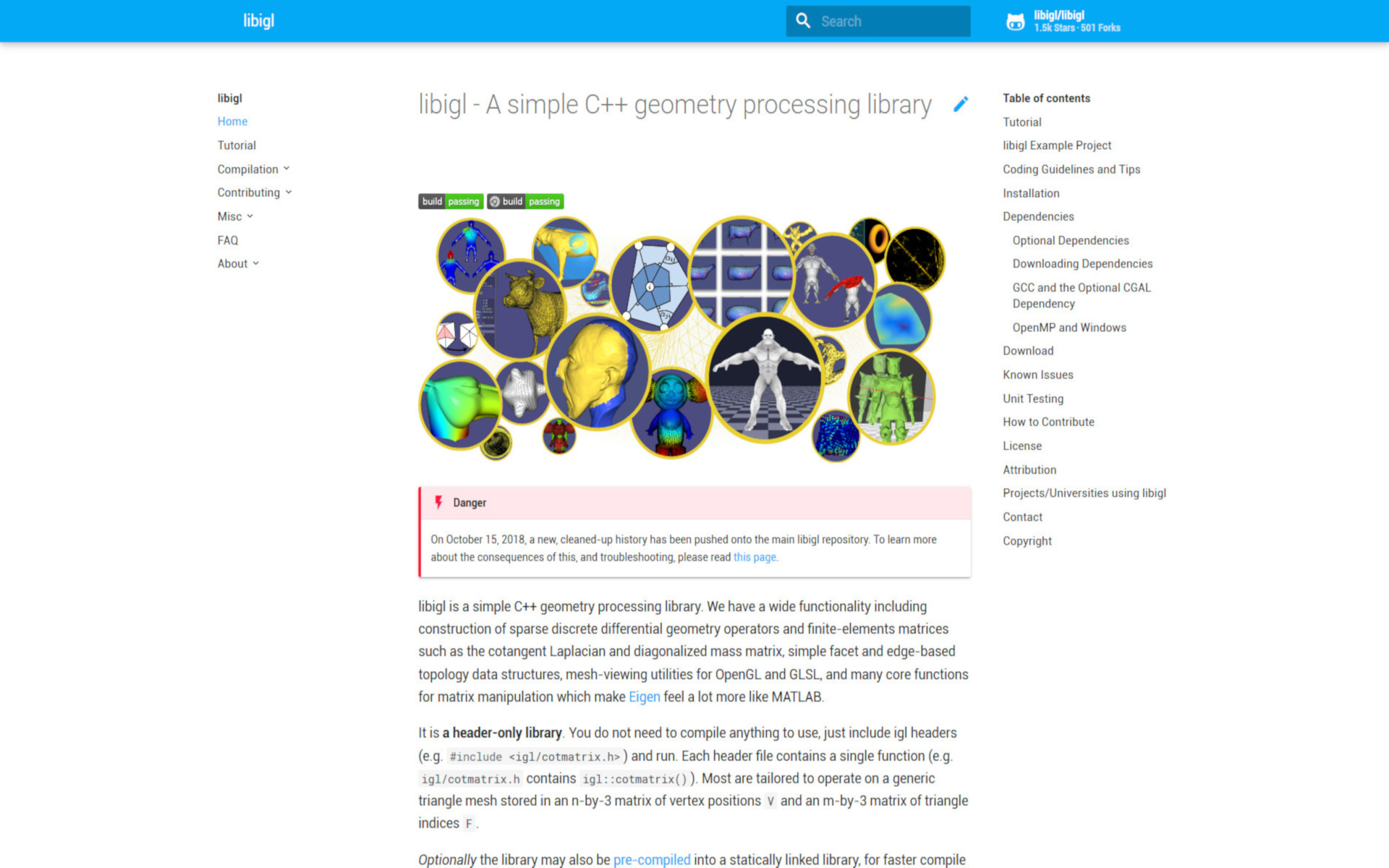libigl - A simple C++ geometry processing library

libigl is a simple C++ geometry processing library. We have a wide functionality including construction of sparse discrete differential geometry operators and finite-elements matrices such as the cotangent Laplacian and diagonalized mass matrix, simple facet and edge-based topology data structures, mesh-viewing utilities for OpenGL and GLSL, and many core functions for matrix manipulation which make Eigen feel a lot more like MATLAB.